+关注继续查看

1.具有自学习功能

2.具有联想存储功能

3.具有高速寻找优化解的能力

# AForge.Net单层网络实现AND运算

AForge.Net中有关神经网络的实现主要在AForge.Neuro中，用install-package AForge.Neuro获取。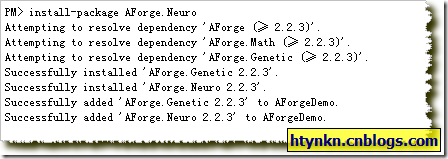1.构建模型

AND运算的话不用多讲，整理一下输入输出：

[0,0]  ===>  

[1,0]  ===>  

[0,1]  ===>  

[1,1]  ===>  

//整理输入输出数据 double[][] input = new double[]; double[][] output = new double[]; input = new double[] { 0, 0 }; output = new double[] { 0 }; input = new double[] { 0, 1 }; output = new double[] { 0 }; input = new double[] { 1, 0 }; output = new double[] { 0 }; input = new double[] { 1, 1 }; output = new double[] { 1 };

2.选择激励函数和学习规则

AForge.Net中的激励函数需实现IActivationFunction 接口，AForge.Net中实现了3种：

BipolarSigmoidFunction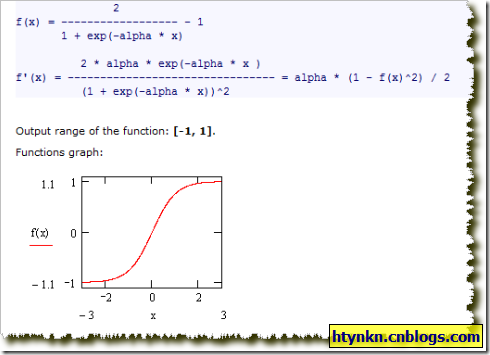SigmoidFunction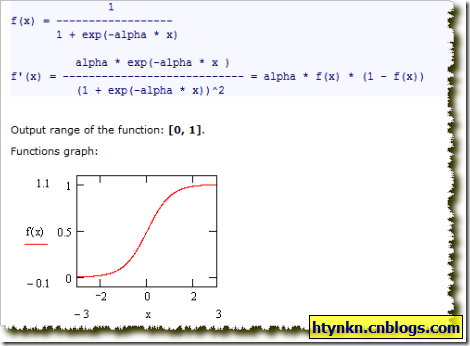ThresholdFunction（阈函数）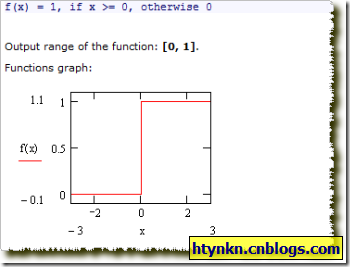AForge.Net中学习函数要实现ISupervisedLearning或者IUnsupervisedLearning接口，程序库实现了5种：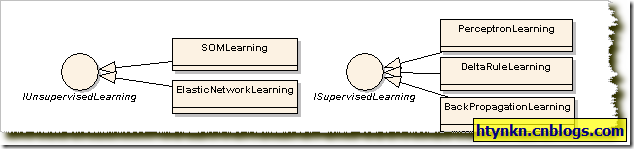//建立网络，层数1，输入2，输出1，激励函数阈函数 ActivationNetwork network = new ActivationNetwork(new ThresholdFunction(), 2, 1);
//学习方法为感知器学习算法 PerceptronLearning teacher = new PerceptronLearning(network);

3.训练网络

teacher.RunEpoch(input, output);

4.获取输出进行处理

//模拟 for (int i = 0; i < 4; i++) {     Console.WriteLine("input{0}:  ===>  {1},{2}  sim{0}:  ===>  {3}", i, input[i], input[i], network.Compute(input[i])); }

//整理输入输出数据 double[][] input = new double[]; double[][] output = new double[]; input = new double[] { 0, 0 }; output = new double[] { 0 }; input = new double[] { 0, 1 }; output = new double[] { 0 }; input = new double[] { 1, 0 }; output = new double[] { 0 }; input = new double[] { 1, 1 }; output = new double[] { 1 };for (int i = 0; i < 4; i++) {     Console.WriteLine("input{0}:  ===>  {1},{2}  output{0}:  ===>  {3}",i,input[i],input[i],output[i]); }//建立网络，层数1，输入2，输出1，激励函数阈函数 ActivationNetwork network = new ActivationNetwork(new ThresholdFunction(), 2, 1);//学习方法为感知器学习算法 PerceptronLearning teacher = new PerceptronLearning(network);//定义绝对误差 double error = 1.0; Console.WriteLine(); Console.WriteLine("learning error  ===>  {0}", error);//输出学习速率 Console.WriteLine(); Console.WriteLine("learning rate ===>  {0}",teacher.LearningRate);//迭代次数 int iterations = 0; Console.WriteLine(); while (error > 0.001) {     error = teacher.RunEpoch(input, output);     Console.WriteLine("learning error  ===>  {0}", error);     iterations++; } Console.WriteLine("iterations  ===>  {0}", iterations); Console.WriteLine(); Console.WriteLine("sim:");//模拟 for (int i = 0; i < 4; i++) {     Console.WriteLine("input{0}:  ===>  {1},{2}  sim{0}:  ===>  {3}", i, input[i], input[i], network.Compute(input[i])); }

# 人工神经网络和模糊系统结合前景

1.逻辑模糊神经网络

2.算术模糊神经网络

3.混合逻辑神经网络45 0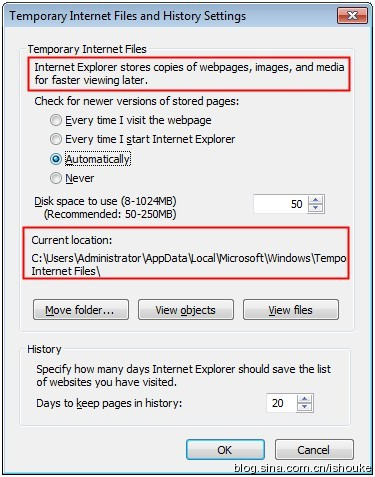41 0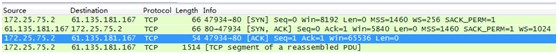42 029 084 040 0227 0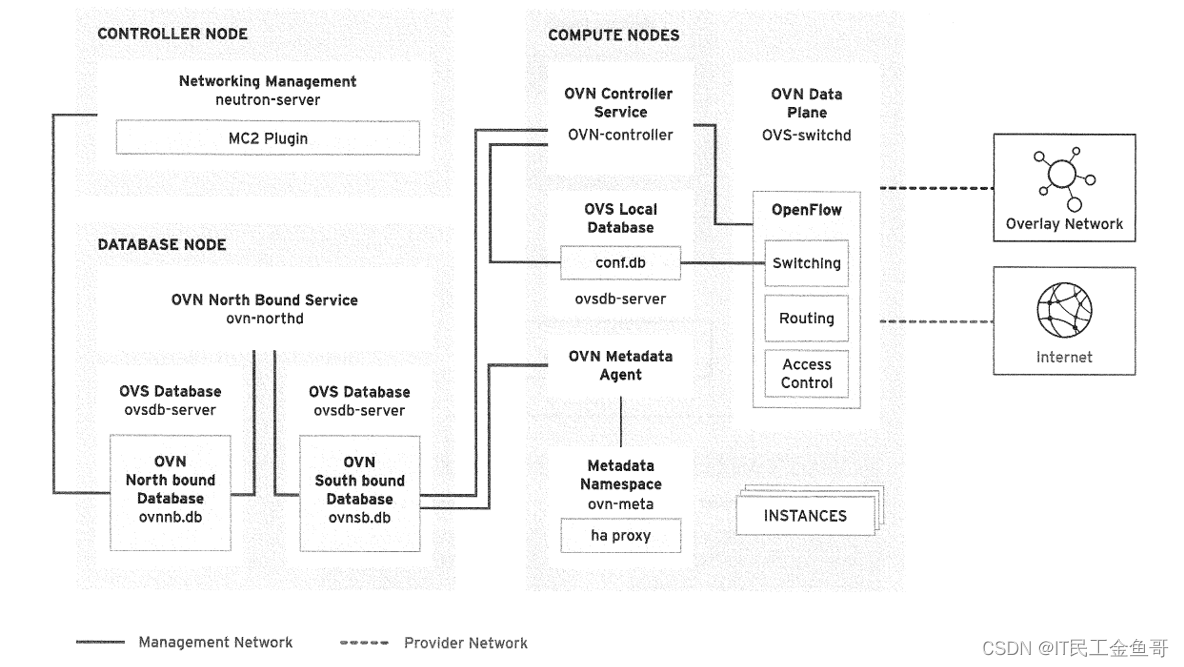670 0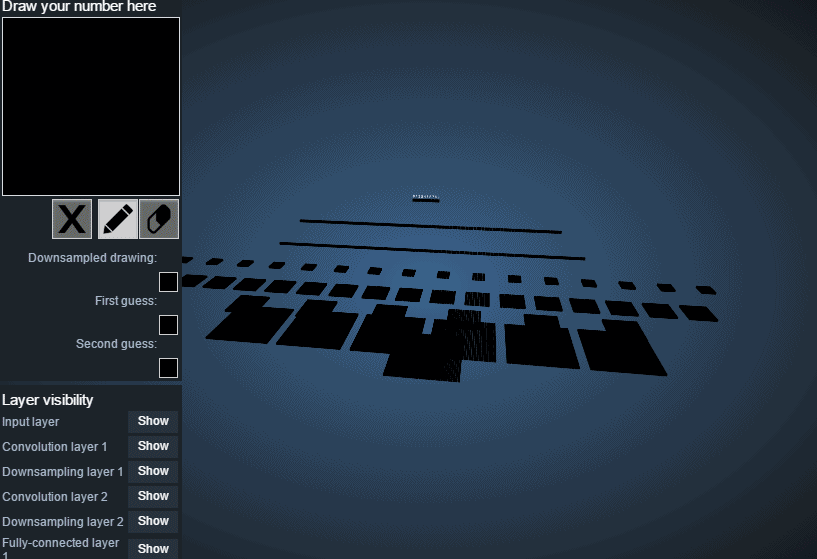DL之CNN：卷积神经网络算法简介之原理简介——CNN网络的3D可视化(LeNet-5为例可视化)
DL之CNN：卷积神经网络算法简介之原理简介——CNN网络的3D可视化(LeNet-5为例可视化)
111 0

【算法实战】7. 集成方法-随机森林656